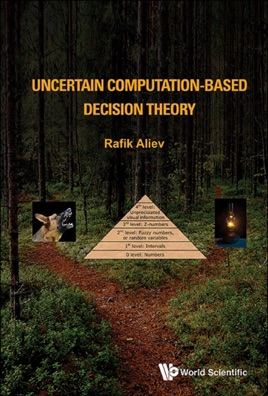• \$139.99

## Publisher Description

Uncertain computation is a system of computation and reasoning in which the objects of computation are not values of variables but restrictions on values of variables.

This compendium includes uncertain computation examples based on interval arithmetic, probabilistic arithmetic, fuzzy arithmetic, Z-number arithmetic, and arithmetic with geometric primitives.

The principal problem with the existing decision theories is that they do not have capabilities to deal with such environment. Up to now, no books where decision theories based on all generalizations level of information are considered. Thus, this self-containing volume intends to overcome this gap between real-world settings' decisions and their formal analysis.
Contents: Decision EnvironmentAnalysis of the Existing Decision TheoriesInterval ComputationProbabilistic ArithmeticFuzzy Type-1 and Fuzzy Type-2 ComputationsComputation with Z-NumbersComputation with U-NumbersFuzzy Geometry Based ComputationsInterval Granular-Based Decision MakingDecision Making in Fuzzy EnvironmentThe Z-Restriction Centered Decision TheorySimulation and Applications